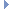OpenMM
TabulatedFunction Class Reference

A TabulatedFunction uses a set of tabulated values to define a mathematical function. More...Inheritance diagram for TabulatedFunction:

List of all members.

## Public Member Functions

def __init__
def Copy
Copy(self) -> TabulatedFunction.

## Detailed Description

A TabulatedFunction uses a set of tabulated values to define a mathematical function.

It can be used by various custom forces.

TabulatedFunction is an abstract class with concrete subclasses for more specific types of functions. There are subclasses for:

• 1, 2, and 3 dimensional functions. The dimensionality of a function means the number of input arguments it takes.
• Continuous and discrete functions. A continuous function is interpolated by fitting a natural cubic spline to the tabulated values. A discrete function is only defined for integer values of its arguments (that is, at the tabulated points), and does not try to interpolate between them. Discrete function can be evaluated more quickly than continuous ones.

## Constructor & Destructor Documentation

 def __init__ ( self, args, kwargs )

## Member Function Documentation

 def Copy ( self )

Copy(self) -> TabulatedFunction.

The documentation for this class was generated from the following file:
• openmm.py